JEE  >  Test: 35 Year JEE Previous Year Questions: Laws of Motion

# Test: 35 Year JEE Previous Year Questions: Laws of Motion

Test Description

## 39 Questions MCQ Test Physics For JEE | Test: 35 Year JEE Previous Year Questions: Laws of Motion

Test: 35 Year JEE Previous Year Questions: Laws of Motion for JEE 2023 is part of Physics For JEE preparation. The Test: 35 Year JEE Previous Year Questions: Laws of Motion questions and answers have been prepared according to the JEE exam syllabus.The Test: 35 Year JEE Previous Year Questions: Laws of Motion MCQs are made for JEE 2023 Exam. Find important definitions, questions, notes, meanings, examples, exercises, MCQs and online tests for Test: 35 Year JEE Previous Year Questions: Laws of Motion below.
Solutions of Test: 35 Year JEE Previous Year Questions: Laws of Motion questions in English are available as part of our Physics For JEE for JEE & Test: 35 Year JEE Previous Year Questions: Laws of Motion solutions in Hindi for Physics For JEE course. Download more important topics, notes, lectures and mock test series for JEE Exam by signing up for free. Attempt Test: 35 Year JEE Previous Year Questions: Laws of Motion | 39 questions in 80 minutes | Mock test for JEE preparation | Free important questions MCQ to study Physics For JEE for JEE Exam | Download free PDF with solutions
 1 Crore+ students have signed up on EduRev. Have you?
Test: 35 Year JEE Previous Year Questions: Laws of Motion - Question 1

### A frame of reference that is accelerated with respect to an inertial frame of reference is called a non-inertial frame of reference. A coordinate system fixed on a circular disc rotating about a fixed axis with a constant angular velocity ω is an example of noninertial frame of reference. The relationship between the force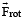experienced by a particle of mass m moving on the rotating disc and the force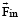experienced by the particle in an inertial frame of reference is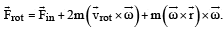where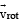is the velocity of the particle in the rotating frame of reference and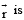is the position vector of the particle with respect to the centre of the disc.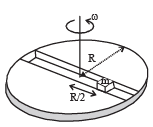Now consider a smooth slot along a diameter of a disc of radius R rotating counter-clockwise with a constant angular speed ω about its vertical axis through its center. We assign a coordinate system with the origin at the center of the disc, the x-axis along the slot, the y-axis perpendicular to the slot and the z-axis along the rotation axis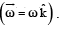A small block of mass m is gently placed in the slot at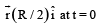and is constrained to move only along the slot. Q. The distance r of the block at time t is

Detailed Solution for Test: 35 Year JEE Previous Year Questions: Laws of Motion - Question 1

Force on the block along slat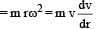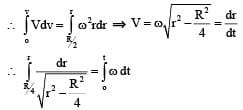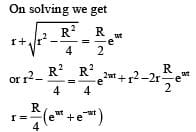Test: 35 Year JEE Previous Year Questions: Laws of Motion - Question 2

### A frame of reference that is accelerated with respect to an inertial frame of reference is called a non-inertial frame of reference. A coordinate system fixed on a circular disc rotating about a fixed axis with a constant angular velocity ω is an example of noninertial frame of reference. The relationship between the forceexperienced by a particle of mass m moving on the rotating disc and the forceexperienced by the particle in an inertial frame of reference iswhereis the velocity of the particle in the rotating frame of reference andis the position vector of the particle with respect to the centre of the disc.Now consider a smooth slot along a diameter of a disc of radius R rotating counter-clockwise with a constant angular speed ω about its vertical axis through its center. We assign a coordinate system with the origin at the center of the disc, the x-axis along the slot, the y-axis perpendicular to the slot and the z-axis along the rotation axisA small block of mass m is gently placed in the slot atand is constrained to move only along the slot. Q. The net reaction of the disc on the block is

Detailed Solution for Test: 35 Year JEE Previous Year Questions: Laws of Motion - Question 2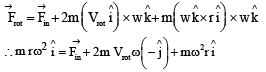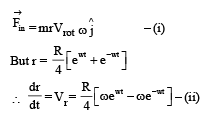Test: 35 Year JEE Previous Year Questions: Laws of Motion - Question 3

### STATEMENT-1 : A cloth covers a table. Some dishes are kept on it. The cloth can be pulled out without dislodging the dishes from the table. STATEMENT-2 : For every action there is an equal and opposite reaction.

Detailed Solution for Test: 35 Year JEE Previous Year Questions: Laws of Motion - Question 3

Statement1 : Cloth can be pulled out with out dislodging the dishes from the table because of inertia.
Therefore, statement – 1 is true.
Statement 2 : This is Newton's third law and hence true. But statement 2 is not a correct explanation of statement 1.

Test: 35 Year JEE Previous Year Questions: Laws of Motion - Question 4

STATEMENT-1 : It is easier to pull a heavy object than to push it on a level ground and

STATEMENT-2 : The magnitude of frictional force depends on the nature of the two surfaces in contact.

Detailed Solution for Test: 35 Year JEE Previous Year Questions: Laws of Motion - Question 4

It is easier to pull a heavy object than to push it on a level ground. Statement-1 is true. This is because the normal reaction in the case of pulling is less as compared by pushing. (f = μ N). Therefore the frictional force is small in case of pulling. statement-2 is true but is not the correct explanation of statement-1.

Test: 35 Year JEE Previous Year Questions: Laws of Motion - Question 5

If a body looses half of its velocity on penetrating 3 cm in a wooden block, then how much will it penetrate more before coming to rest?

Detailed Solution for Test: 35 Year JEE Previous Year Questions: Laws of Motion - Question 5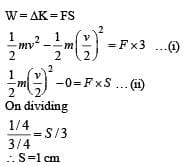Test: 35 Year JEE Previous Year Questions: Laws of Motion - Question 6

A lift is moving down with acceleration a. A man in the lift drops a ball inside the lift. The acceleration of the ball as observed by the man in the lift and a man standing stationary on the ground are respectively

Detailed Solution for Test: 35 Year JEE Previous Year Questions: Laws of Motion - Question 6

For the man standing in the left, the acceleration of   the ball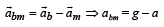Where 'a' is the acceleration of the mass (because the acceleration of the lift is 'a')

For the man standing on the ground, the acceleration of the ball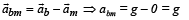Test: 35 Year JEE Previous Year Questions: Laws of Motion - Question 7

When forces F1, F2, F3 are acting on a particle of mass m such that F2 and F3 are mutually perpendicular, then the particle remains stationary. If the force F1 is now removed then the acceleration of the particle is

Detailed Solution for Test: 35 Year JEE Previous Year Questions: Laws of Motion - Question 7

When F1 , F2 and F3 are acting on a particle then the particle remains stationary. This means that the resultant of F1 F2 and F3 is zero. When F1 is removed, F2 and F3 will remain. But the resultant of F2 and F3 should be equal and opposite to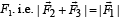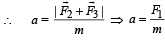Test: 35 Year JEE Previous Year Questions: Laws of Motion - Question 8

Two forces are such that the sum of their magnitudes is 18 N and their resultant is 12 N which is perpendicular to the smaller force. Then the magnitudes of the forces are

Detailed Solution for Test: 35 Year JEE Previous Year Questions: Laws of Motion - Question 8

Let the two forces be F1 and F2 and let F2 < F1 . R is the resultant force.

Given F1 + F2 = 18 ...(i)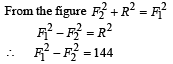Only option (b) follows equation (i) and (ii).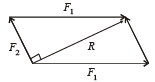Test: 35 Year JEE Previous Year Questions: Laws of Motion - Question 9

Speeds of two identical cars are u and 4u at the specific instant. The ratio of the respective distances in which the two cars are stopped from that instant is

Detailed Solution for Test: 35 Year JEE Previous Year Questions: Laws of Motion - Question 9

ΔK = FS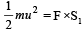… (i)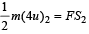...(ii)

Dividing (i) and (ii),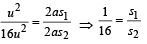Test: 35 Year JEE Previous Year Questions: Laws of Motion - Question 10

A light string passing over a smooth light pulley connects two blocks of masses m1 and m2 (vertically). If the acceleration of the system is g/8, then the ratio of the masses is

Detailed Solution for Test: 35 Year JEE Previous Year Questions: Laws of Motion - Question 10

For mass m1
m1g – T = m1a
For mass m2
T–m2g = m2a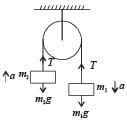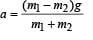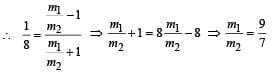Test: 35 Year JEE Previous Year Questions: Laws of Motion - Question 11

Three identical blocks of masses m = 2 kg are drawn by a force F = 10.2 N with an acceleration of 0. 6 ms–2 on a frictionless surface, then what is the tension (in N) in the string between the blocks B and C?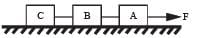Detailed Solution for Test: 35 Year JEE Previous Year Questions: Laws of Motion - Question 11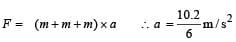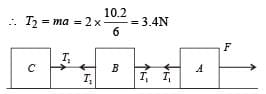Test: 35 Year JEE Previous Year Questions: Laws of Motion - Question 12

One end of a massless rope, which passes over a massless and frictionless pulley P is tied to a hook C while the other end is free. Maximum tension that the rope can bear is 360 N. With what value of maximum safe acceleration (in ms–2) can a man of 60 kg climb on the rope?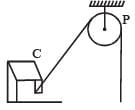Detailed Solution for Test: 35 Year JEE Previous Year Questions: Laws of Motion - Question 12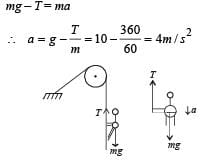Test: 35 Year JEE Previous Year Questions: Laws of Motion - Question 13

A spring balance is attached to the ceiling of a lift. A man hangs his bag on the spring and the spring reads 49 N, when the lift is stationary. If the lift moves downward with an acceleration of 5 m/s2, the reading of the spring balance will be

Detailed Solution for Test: 35 Year JEE Previous Year Questions: Laws of Motion - Question 13

For the bag accelerating down mg – T = ma

∴ T= m( g –a)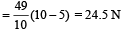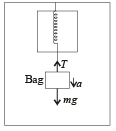Test: 35 Year JEE Previous Year Questions: Laws of Motion - Question 14

Three forces start acting simultaneously on a particle moving with velocity,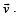These forces are represented in magnitude and direction by the three sides of a triangle  ABC. The particle will now move with velocity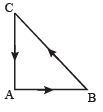Detailed Solution for Test: 35 Year JEE Previous Year Questions: Laws of Motion - Question 14

As shown in the figure, the three forces are represented by the sides of a triangle taken in the same order. Therefore the resultant force is zero.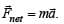Therefore acceleration is also zero ie velocity remains unchanged.

Test: 35 Year JEE Previous Year Questions: Laws of Motion - Question 15

A horizontal force of 10 N is necessary to just  hold a block stationary against a wall. The coefficient of friction between the block and the wall is 0.2. The weight of the block  is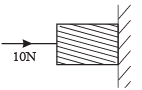Detailed Solution for Test: 35 Year JEE Previous Year Questions: Laws of Motion - Question 15

For the block to remain stationary with the wall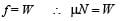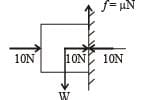0.2 × 10 = W ⇒ W= 2N

Test: 35 Year JEE Previous Year Questions: Laws of Motion - Question 16

A marble block of mass 2 kg lying on ice when given a velocity of 6 m/s is stopped by friction in 10 s. Then the coefficient of  friction is

Detailed Solution for Test: 35 Year JEE Previous Year Questions: Laws of Motion - Question 16

u = 6 m/s, v = 0, t = 10s,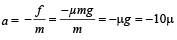v = u + at
0 = 6 – 10
µ × 10
∴  µ = 0.06

Test: 35 Year JEE Previous Year Questions: Laws of Motion - Question 17

A block of  mass M is pulled along a horizontal frictionless surface by a rope of mass m. If a force P is applied at the free end of the rope, the force exerted by the rope on the block is

Detailed Solution for Test: 35 Year JEE Previous Year Questions: Laws of Motion - Question 17

Taking the rope and the block as a system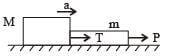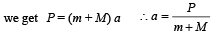Taking the block as a system, we get  T = Ma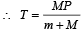Test: 35 Year JEE Previous Year Questions: Laws of Motion - Question 18

A light spring balance hangs from the hook of the other light spring balance and a block of mass M kg hangs from the former one. Then the true statement about the scale reading is

Detailed Solution for Test: 35 Year JEE Previous Year Questions: Laws of Motion - Question 18

The Earth pulls the block by a force Mg. The block in turn exerts a force Mg on the spring of spring balance S1 which therefore shows a reading of M kgf.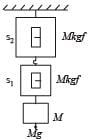The spring S1 is massless. Therefore it exerts a force of Mg on the spring of spring balance S2 which shows the reading of M kgf.

Test: 35 Year JEE Previous Year Questions: Laws of Motion - Question 19

A rocket with  a lift-off mass 3.5 × 104 kg is blasted upwards with an initial acceleration of 10m/s2. Then the initial thrust of the blast is

Detailed Solution for Test: 35 Year JEE Previous Year Questions: Laws of Motion - Question 19

As shown in the figure F – mg = ma

∴ F = m (g + a)
= 3.5 × 104 (10+10)
= 7 × 105 N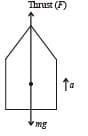Test: 35 Year JEE Previous Year Questions: Laws of Motion - Question 20

Two masses m1 = 5 kg and m2 = 4.8 kg tied  to a string are hanging over a light frictionless pulley. What is the acceleration of the masses when left free to move ?

(g = 9.8m / s2)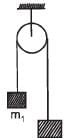Detailed Solution for Test: 35 Year JEE Previous Year Questions: Laws of Motion - Question 20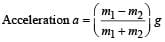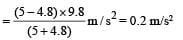Test: 35 Year JEE Previous Year Questions: Laws of Motion - Question 21

A block rests on a rough inclined plane making an angle of 30° with the horizontal. The coefficient of static friction between the block and the plane is 0.8. If the frictional force on the block is 10 N, the mass of the block (in kg) is

(take g = 10 m /s2)

Detailed Solution for Test: 35 Year JEE Previous Year Questions: Laws of Motion - Question 21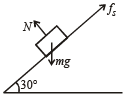mg sinθ = fs (for body to be at rest)

⇒ m x10 x sin 30°= 10
⇒ m x 5 = 10 ⇒ m = 2.0 kg

Test: 35 Year JEE Previous Year Questions: Laws of Motion - Question 22

A smooth block is released at rest on a 45° incline and then slides a distance ‘d’. The time taken to slide is ‘n’ times as much to slide on rough incline than on a smooth incline.
The coefficient of friction is

Detailed Solution for Test: 35 Year JEE Previous Year Questions: Laws of Motion - Question 22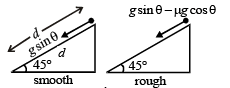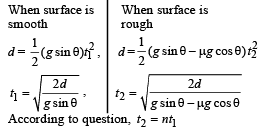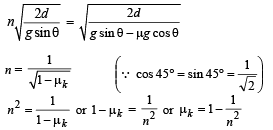Test: 35 Year JEE Previous Year Questions: Laws of Motion - Question 23

A parachutist after bailing out falls 50 m without friction.
Wh en par achute open s, it decelerates at 2 m /s2 . He reaches the ground with a speed of 3 m/s. At what height, did he bail out ?

Detailed Solution for Test: 35 Year JEE Previous Year Questions: Laws of Motion - Question 23

The velocity of parachutist when parachute opens is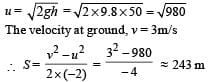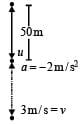Initially he has fallen 50 m.
∴ Total height from where he bailed out = 243 + 50 = 293 m

Test: 35 Year JEE Previous Year Questions: Laws of Motion - Question 24

A bullet fired into a fixed target loses half of its velocity after penetrating 3 cm. How much further it will penetrate before coming to rest assuming that it faces constant resistance to motion ?

Detailed Solution for Test: 35 Year JEE Previous Year Questions: Laws of Motion - Question 24

Let K be the initial kinetic energy and F be the resistive force. Then according to work-energy theorem,

W = ΔK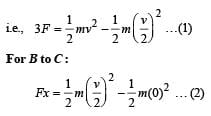Dividing eqns. (1) and (2) we get x/3 = 1/3

or  x = 1 cm

Test: 35 Year JEE Previous Year Questions: Laws of Motion - Question 25

An annular ring with inner and outer radii R1 and R2 is rolling without slipping with a uniform angular speed. The ratio of the forces experienced by the two particles situated on the inner and outer parts of the ring,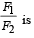Detailed Solution for Test: 35 Year JEE Previous Year Questions: Laws of Motion - Question 25

Force experienced by the particle, F = mω2R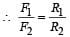Test: 35 Year JEE Previous Year Questions: Laws of Motion - Question 26

The upper half of an inclined plane with inclination φ is perfectly smooth while the lower half is rough. A body starting from rest at the top will again come to rest at the bottom if the coefficient of friction for the lower half is given by

Detailed Solution for Test: 35 Year JEE Previous Year Questions: Laws of Motion - Question 26

According to work-energy theorem, W = ΔK = 0 (Since initial and final speeds are zero)

∴ Workdone by friction + Work done by gravity = 0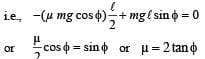Test: 35 Year JEE Previous Year Questions: Laws of Motion - Question 27

A particle of mass 0.3 kg subject to a force F = – kx with k = 15 N/m . What will be its initial acceleration if it is released from a point 20 cm away from the origin ?

Detailed Solution for Test: 35 Year JEE Previous Year Questions: Laws of Motion - Question 27

Mass (m) = 0.3 kg  ⇒ F = m.a = 15x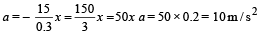Test: 35 Year JEE Previous Year Questions: Laws of Motion - Question 28

A block is kept on a frictionless inclined surface with angle of inclination ‘α’ . The incline is given an acceleration ‘a’ to keep the block stationary. Then a  is equal to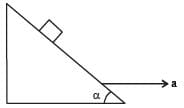Detailed Solution for Test: 35 Year JEE Previous Year Questions: Laws of Motion - Question 28

From diagram,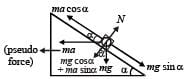For block to remain stationary,

mg sin α = ma cosa ⇒ a = g tan α

Test: 35 Year JEE Previous Year Questions: Laws of Motion - Question 29

Consider a car moving on a straight road with a speed of 100 m/s . The distance at which car can be stopped is [μk = 0.5]

Detailed Solution for Test: 35 Year JEE Previous Year Questions: Laws of Motion - Question 29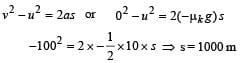Test: 35 Year JEE Previous Year Questions: Laws of Motion - Question 30

A mass of M kg is suspended by a weightless string. The horizontal force that is required to displace it until the string makes an angle of 45° with the initial vertical direction is

Detailed Solution for Test: 35 Year JEE Previous Year Questions: Laws of Motion - Question 30

Work done by tension + Work done by force (applied) + Work done by gravitational force = change in kinetic energy

Work done by tension is zero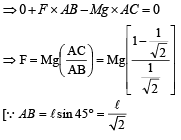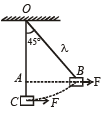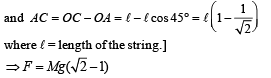Test: 35 Year JEE Previous Year Questions: Laws of Motion - Question 31

A ball of mass 0.2 kg is thrown vertically upwards by applying a force by hand. If the hand moves 0.2 m while applying the force and the ball goes upto 2 m height further, find the magnitude of the force. (Consider g = 10 m/s2).

Detailed Solution for Test: 35 Year JEE Previous Year Questions: Laws of Motion - Question 31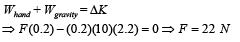Test: 35 Year JEE Previous Year Questions: Laws of Motion - Question 32

A player caught a cricket ball of mass 150 g moving at a rate of 20 m/s. If the catching process is completed in 0.1s, the force of the blow exerted by the ball on the hand of the player is equal to

Detailed Solution for Test: 35 Year JEE Previous Year Questions: Laws of Motion - Question 32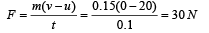Test: 35 Year JEE Previous Year Questions: Laws of Motion - Question 33

A coin is placed on a horizontal platform which undergoes vertical simple harmonic motion of angular frequency w. The amplitude of oscillation is gradually increased. The coin will leave contact with the platform for the first time (a) at the mean position of the platform

Detailed Solution for Test: 35 Year JEE Previous Year Questions: Laws of Motion - Question 33

For block A to move in SHM.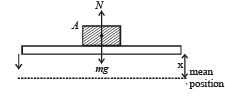mg – N = mω2x

where x is the distance from mean position For block to leave contact N = 0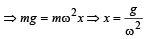Test: 35 Year JEE Previous Year Questions: Laws of Motion - Question 34

A block of mass m is connected to another block of mass M by a spring (massless) of spring constant k. The block are kept on a smooth horizontal plane. Initially the blocks are at rest and the spring is unstretched. Then a constant force F starts acting on the block of mass M to pull it. Find the force of the block of mass m.

Detailed Solution for Test: 35 Year JEE Previous Year Questions: Laws of Motion - Question 34

Drawing free body-diagrams for m & M,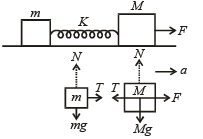we get  T = ma  and F – T = Ma where T is force due to spring
⇒ F – ma = Ma  or,, F = Ma + ma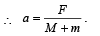Now, force acting on the block of mass m is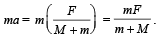Test: 35 Year JEE Previous Year Questions: Laws of Motion - Question 35

Two fixed frictionless inclined planes making an angle 30° and 60° with the vertical are shown in the figure. Two blocks A and B are placed on the two planes. What is the relative vertical acceleration of A with respect to B ?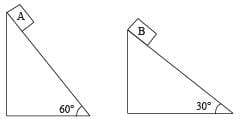Detailed Solution for Test: 35 Year JEE Previous Year Questions: Laws of Motion - Question 35

mg sin θ = ma ∴ a = g sin θ where a is along the inclined plane
∴ vertical component of acceleration is g sin2 θ
∴ relative vertical acceleration of A with respect to B is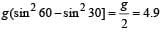m /s2 in vertical direction

Test: 35 Year JEE Previous Year Questions: Laws of Motion - Question 36

A mass ‘m’ is supported by a massless string wound around a uniform hollow cylinder of mass m and radius R. If the string does not slip on the cylinder, with what acceleration will the mass fall or release?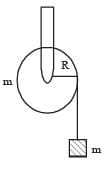Detailed Solution for Test: 35 Year JEE Previous Year Questions: Laws of Motion - Question 36

From figure,

Acceleration a = Rα …(i)
and mg – T = ma …(ii)
From equation (i) and (ii)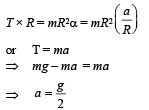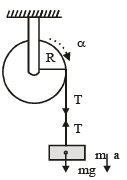Test: 35 Year JEE Previous Year Questions: Laws of Motion - Question 37

A block of mass m is placed on a surface with a vertical cross section given by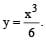If the coefficient of friction is 0.5, the maximum height above the ground at which the block can be placed without slipping is:

Detailed Solution for Test: 35 Year JEE Previous Year Questions: Laws of Motion - Question 37

At limiting equilibrium, μ = tan θ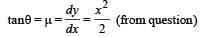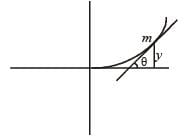∵ Coefficient of friction μ = 0.5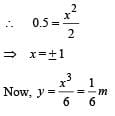Test: 35 Year JEE Previous Year Questions: Laws of Motion - Question 38

Given in the figure are two blocks A and B of weight 20 N and 100 N, respectively. These are being pressed against a wall by a force F as shown. If the coefficient of friction between the blocks is 0.1 and between block B and the wall is 0.15, the frictional force applied by the wall on block B is: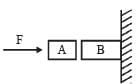Detailed Solution for Test: 35 Year JEE Previous Year Questions: Laws of Motion - Question 38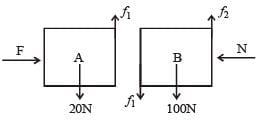Assuming both the blocks are stationary N = F
f1 = 20 N
f2 = 100 + 20 = 120N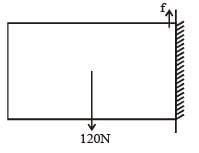Considering the two blocks as one system and due to equilibrium f = 120N

Test: 35 Year JEE Previous Year Questions: Laws of Motion - Question 39

A point particle of mass m, moves long the uniformly rough track PQR as shown in the figure. The coefficient of friction, between the particle and the rough track equals μ. The particle is released, from rest from the point P and it comes to rest at a point R. The energies, lost by the ball, over the parts, PQ and QR, of the track, are equal to each other, and no energy is lost when particle changes direction from PQ to QR.

The value of the coefficient of friction μ and the distance x (= QR), are, respectively close to :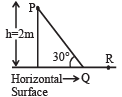Detailed Solution for Test: 35 Year JEE Previous Year Questions: Laws of Motion - Question 39

Loss in P.E. = Work done against friction from p →Q + work done against friction from Q → R
mgh = μ(mg cosθ) PQ + μmg (QR)
h = μ cos θ × PQ + m(QR)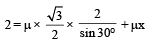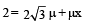--- (i)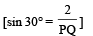Also work done P → Q = work done Q → R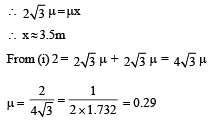## Physics For JEE

258 videos|633 docs|256 tests
 Use Code STAYHOME200 and get INR 200 additional OFF Use Coupon Code
Information about Test: 35 Year JEE Previous Year Questions: Laws of Motion Page
In this test you can find the Exam questions for Test: 35 Year JEE Previous Year Questions: Laws of Motion solved & explained in the simplest way possible. Besides giving Questions and answers for Test: 35 Year JEE Previous Year Questions: Laws of Motion, EduRev gives you an ample number of Online tests for practice

## Physics For JEE

258 videos|633 docs|256 tests